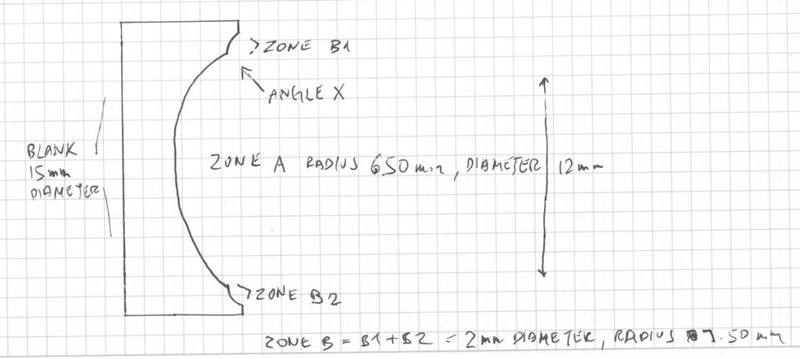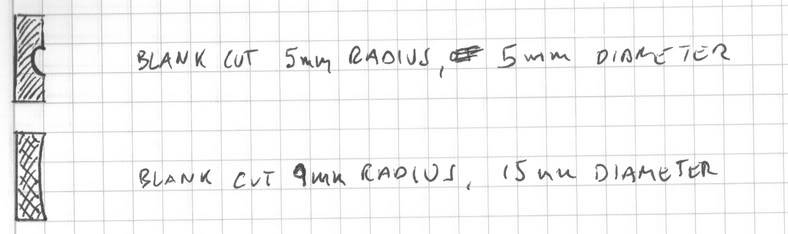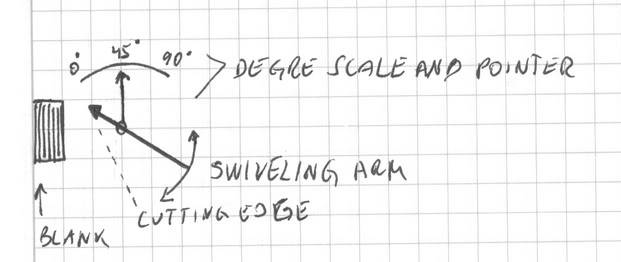# Diameter into angles

The manual lathe I work on has a degree scale for the arm. I have the radiuses in mm and the diameters in mm of two zones I have to cut. What formula can I use to determine the angle which delimits the two zones?

For example:

Say I have an item 15mm in diameter. Inner zone A is to have a radius of 6.50mm and a diameter of 12mm. Outer zone B is to have a radius of 7.50mm and diameter of 2mm. I cut zone A first covering perhaps 14.5 mm diameter. Next I cut zone B. How can I determine at which angle to stop the arm when I'm cutting zone B so that both zones are the correct diameter?

First of all, it seems that you have some numbers stated incorrectly (unless I'm not understanding your statement - perhaps a diagram would help): you state that zone A has a radius of 6.5mm and a diameter of 12mm; since diameter is twice the radius, don't you mean "a diameter of 13mm?" Also, zone B has a radius of 7.5mm and a diameter of 2mm? How is that possible?

I believe you would first need to know the length of the arm as measured from the center of its pivot point to the tip of the cutting edge (which, presumably, would be the same distance as measured from the center of the cutting arm's pivot point to the center of rotation of the spindle).

Since the cutting arm swings in an angular motion, that means that the path of the cutting blade describes an arc. So, if you want to cut, say, 3" of material, the blade would travel 3" in arc length.

Then you could use the formula

$$A=\frac{180 D}{\pi R}$$

Where A is the angle you are looking for (the arc length), D is the distance the blade needs to travel (the amount to cut off), and R is the radius of the cutting arm.

So, suppose the cutting arm measured 20" from its pivot point to the tip of the cutting blade, but since you are working in millimeters, we have 20in * 25.4mm/in = 508mm (R = 508). Then from your example, the item is 15mm in diameter (a radius of 7.5mm) and you want to cut "zone A" down to a radius of 6.5mm, you want to cut off 1mm of material, therefore D = 1. Plugging into the formula, you have:

$$A=\frac{180 \times 1}{\pi \times 508} \approx 0.113^\circ$$

Hope that helps!

Thank you for the help. Let me see if I can clarify things a bit.

Here's a pic if the example.On the lathe I can shorten and lengthen the distance of the cutting edge to the center of the pivot. The gauge that measures that distance gives me a reading in mm of the radius at which it will cut the blank. The smaller the distance, the smaller the radius. The larger the distance, the larger the radius.

In practice I can cut any radius within a range of 4mm-14mm any diameter as long as it doesn't exceed the diameter of the blank of course. For example, I can cut a diameter of 5mm in a radius of 5mm, or 15mm in a radius of 9mm. So somehow radius and diameter are independent of each other.The degree scale on the lathe goes from 0 - 90. Actual cutting takes place between 45 and 0 so the angle X has to be somewhere between 0-45 degrees. Since Zone B will always be smaller than Zone A, the angle has usually been in the 30s when I've done it by eye. But the angle changes as the radius of Zone B changes so it's impossible to do it by eye and get it right every time, since in practice, the diameter is measured up to hundredths of mm.

I was going to suggest using a ball-nosed end mill and a rotary table for this, but I can't find 6.5mm or 7.5mm mills. You really need a CNC lathe to accurately machine this type of geometry.